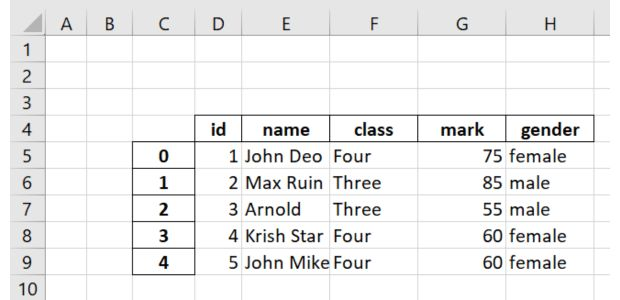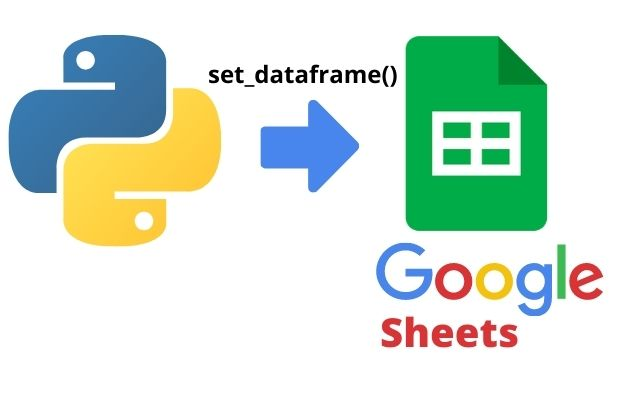# to_excel(): DataFrame to Excel

Create Excel file using Pandas DataFrame .
``````import pandas as pd
my_dict={
'NAME':['Ravi','Raju','Alex'],
'ID':[1,2,3],'MATH':[30,40,50],
'ENGLISH':[20,30,40]
}
df = pd.DataFrame(data=my_dict) # DataFrame from dicitionary
df.to_excel('D:\my_file.xlsx') # create Excel file ``````
to_excel(): Data from Pandas DataFrame to Excel file #B02

Options

## index: bool, default=True

By default pandas will add one index column. We can remove index by using option index=False
``df.to_excel('D:\my_file.xlsx',index=False)``

## Storing Path

We can keep in D drive ( root )
``df.to_excel('D:\my_file.xlsx')``
Inside data directory
``df.to_excel('D:\data\my_file.xlsx')``

## na_rep Blank data

How to handle if data is blank, we can use na_rep='*'
``df.to_excel('D:\my_file.xlsx',na_rep='*')``

## columns

List of columns to use while creating Excel file. Use only id and name columns here
``df2.to_excel('C:\\data\\student2.xlsx', columns=['id','name'])``

Aliases for the column names we can use. WE created a list with our headers and then used the same.
``````my_heads=['my_id','my_names','my_class','my_mark','my_gender']

## startrow

Default value is 0, this is the top row.

## startcol

Default value is 0, this is the left most column.
``df2.to_excel('C:\\data\\student2.xlsx',startrow=3,startcol=2)``## engine

We can use 'openpyxl' or 'xlsxwriter'

## freeze_panes

A tuple , one-based bottommost row and rightmost column that is to be frozen.
``df2.to_excel('C:\\data\\student2.xlsx',freeze_panes=(1,5))``

## float_format

Formatting the floating point numbers. `float_format="%.2f"` will update the value 0.1234 to 0.12

## Storing different worksheets

Excel has worksheets to store data in different sheets.
When we create a new excel file the data stored in Sheet1. We can create different Sheets and store data in different worksheets.

By using sheet_name we can store in worksheet with specific name sheet_name='my_Sheet_1'
``df.to_excel('D:\my_file.xlsx',index=False,sheet_name='my_Sheet_1')``
This will store our data in my_sheet_1 worksheet of file name my_file.xlsx at root of D drive.

## multiple worksheets

We will use one object of ExcelWriter to create multiple worksheets in a excel file.
``````import pandas as pd
my_dict={
'NAME':['Ravi','Raju','Alex'],
'ID':[1,2,3],'MATH':[30,40,50],
'ENGLISH':[20,30,40]
}
df = pd.DataFrame(data=my_dict)
df2 = df.copy() # copy of df

with pd.ExcelWriter('D:my_file.xlsx') as my_excel_obj: #Object created
df.to_excel(my_excel_obj,sheet_name='my_Sheet_1')
df2.to_excel(my_excel_obj,sheet_name='my_Sheet_2')``````
Above code will create excel file with two worksheets. Here the new file name is my_file.xlsx with two worksheets.

## Appending worksheets

We will add two more worksheets to the existing files by opening the file in append mode.

Note that we are using the same my_file.xlsx file created in above code.

We will be using mode='a' and engine='openpyxl' while creating the ExcelWriter object.
``````import pandas as pd
my_dict={
'NAME':['Ravi','Raju','Alex'],
'ID':[1,2,3],'MATH':[30,40,50],
'ENGLISH':[20,30,40]
}
df = pd.DataFrame(data=my_dict)
df2 = df.copy() # copy of df

with pd.ExcelWriter('D:my_file.xlsx',mode='a',engine='openpyxl') as my_excel_obj:
df.to_excel(my_excel_obj,sheet_name='my_Sheet_3')
df2.to_excel(my_excel_obj,sheet_name='my_Sheet_4')``````
This code will add two more worksheets my_Sheet_3 and my_Sheet_4 to existing workbook my_file.xlsx .

While executing the above code, you may get error saying Append mode is not supported with xlsxwriter. To solve this issue use engine='openpyxl' while creating the ExcelWriter object.

## Data from MySQL table

We can read data from MySQL table and then store them in excel file.
``````import mysql.connector
import pandas as pd
my_connect = mysql.connector.connect(
host="localhost",
user="userid",
database="my_tutorial"
)
####### end of connection ####
sql="SELECT * FROM student "

df.to_excel('D:\my_file.xlsx')``````
In above code we have first connected to MySQL database and then collected the records of student table by using read_sql() to a DataFrame. Finally we saved them in an excel file using to_excel().

## Using SQLAlchemy MySQL connection

Read more on MySQL with SQLAlchemy connection. Below code will create student.xlsx file in the same directory, you can add path ( as above ) if you want the file to be created at different location.
``````import pandas as pd
from sqlalchemy import create_engine
my_conn = create_engine("mysql+mysqldb://userid:pw@localhost/my_db")
sql="SELECT * FROM student "
We can collect data of different classes from our student table and store them in one excel file by keeping in different worksheets. So all students of class Four will be in one worksheet named as Four and similarly another worksheet for class Three students. You can extend this to other classes also.
``````import pandas as pd
from sqlalchemy import create_engine
my_conn = create_engine("mysql+mysqldb://userid:pw@localhost/my_db")
sql="SELECT * FROM student WHERE class='Three'"
sql2="SELECT * FROM student WHERE class='Four'"
with pd.ExcelWriter('D:\\my_data\\student.xlsx',engine='openpyxl') as my_obj:
df.to_excel(my_obj,sheet_name='Three')
df2.to_excel(my_obj,sheet_name='Four')``````

## Storing part of the data

We can filter the DataFrame and then save the rows in xlsx file. For this we will use our test.csv file as we have more rows.
Now let us store only two columns, class and name
``````import pandas as pd
df=df.loc[:,['class','name']]
df = pd.DataFrame(data=df)
df.to_excel('my_file.xlsx',index=False)``````
We can use various other filters to manage the data and store in CSV file. You can rad more on filters sections.

## Inserting DataFrame to google sheets## Questions

Subscribe to our YouTube Channel here

## Subscribe

* indicates required
Subscribe to plus2netplus2net.com